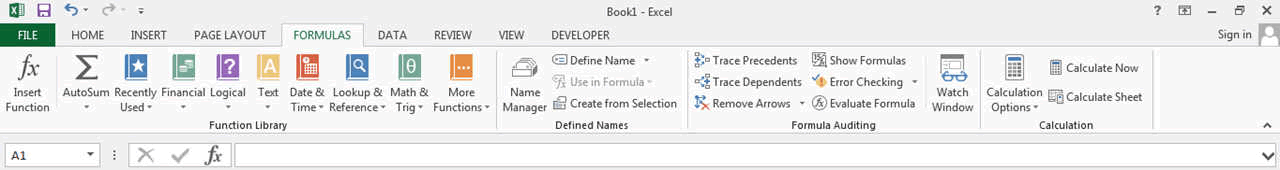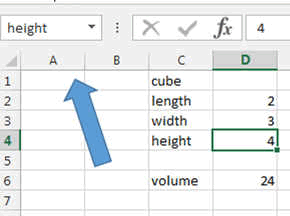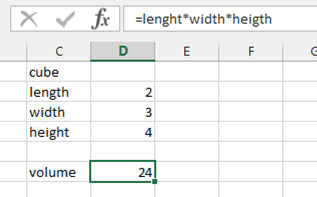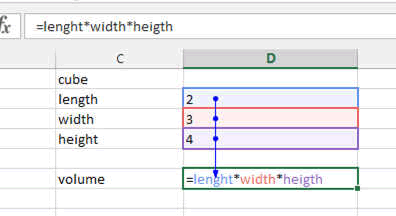## The Formulas Ribbon

The ribbon is a visual solution from Microsoft to put our hands on all the functions needed to create. The term Menu is in principle no longer used.

On a tablet or Microsoft surface, the ribbon are moved laterally with finger or stylus.

The FORMULAS ribbon will be very useful if you decide to go into very complex worksheets and use many different formulas. But if you keep simple and follow the KISS principle than no worries, you wont need this Ribbon so much.

If you need specific formulas, then this is very useful because all the formulas are sorted according to their use: recently used ones, scientific, financial, logical or related to time and date or math and trig.... there are so many....

Here a quick overview.The Function Library is the main part here and allows you to find the right formula according to its use.

The Defined Names is very useful because in Excel you can give a name to cells. Like here we called the cells "length, width and height" to calculate the volume we just need to write volume = length x width x height.
The defined name area allows you to manage all the variables or names you have used in your various spreadsheets.The Formula Auditing area allows you to check your formulas. It shows with arrows the various cells who are involved in a formula. Great USE!!

The watch windows allows you to set up a small window where you can enter all the cells you want to watch simultaneously.The Calculation area allows you to block or unblock the calculation. For example if you want to change different values in a formula but do not want to see the end result change until you decide so, then this is where it is done.